# CBSE Solutions for Class 10 Maths

#### Select CBSE Solutions for class 10 Subject & Chapters Wise :

Find the common difference of the AP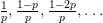. (2013D)

Answer :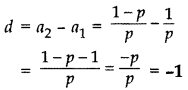Find the common difference of the A.P.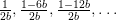. (2013D)

Answer :

d = a2 – a1 =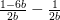=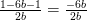= -3

Find the common difference of the A.P.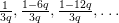. (2013D)

Answer :

d = a2 – a1 =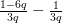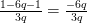= -2

Calculate the common difference of the A.P.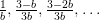. (2013D)

Answer :

d = a2 – a1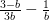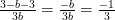Calculate the common difference of the A.P.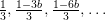(2013OD)

Answer :

d = a2 – a1 =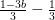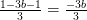= -b

What is the common difference of an A.P. in which a21 – a7 = 84? (2017OD )

Answer :

a21 – a7 = 84 …[Given in the question
∴ (a + 20d) – (a + 6d) = 84 …[an = a + (n – 1)d
20d – 6d = 84
14d = 84 ⇒ d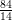= 6

Find the 9th term from the end (towards the first term) of the A.P.    5,9,13, …, 185. (2016D)

Answer :

Here, a = 5, d = 9 – 5 = 4, l = 185
9th term from the end = 185 – (9 – 1)4
= 185 – 8 × 4 = 185 – 32 = 153

The angles of a triangle are in A.P., the least being half the greatest. Find the angles. (2011D)

Answer :

40°, 60°, 80°

Is -150  a term of the A.P. 17, 12, 7, 2, … ? (2011D)

Answer :

no

Which term of the progression 4, 9, 14, 19, … is 109? (2011D)

Answer :

22nd term

If pth, qth and rth terms of an A.P. are a, b, c respectively, then show that (a – b)r + (b – c)p+ (c – a)q = 0. (2011D)

Answer :

Let A be the first term and D be the common difference of the given A.P.
pth term = A + (p – 1)D = a …(A)
qth term = A + (q – 1)D = b …(B)
rth term = A + (r – 1)D = c … (C)
L.H.S. = (a – b)r + (b – c)p + (c – a)q
= [A + (p – 1)D – (A + (q – 1)D)]r + [A + (q – 1)D – (A + (r – 1)D)]p + [A + (r – 1)D – (A + (p – 1)D)]q
= [(p – 1 – q + 1)D]r + [(q – 1 – r + 1)D]p + [(r – 1 – p + 1)D]q
= D[(p – q)r + (q – r)p + (r – p)q]
= D[pr – qr + qp – rp + rq – pq]
= D = 0 = R.H.S.

The 17th term of an AP is 5 more than twice its 8th term. If the 11th term of the AP is 43, then find its nth term. (2012D)

Answer :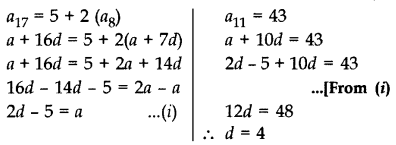From (i),
a = 2(4) – 5 = 8 – 5 = 3
As an = a + (n – 1) d
an = 3 + (n – 1) 4 = 3 + 4n – 4
an = (4n – 1)

The 15th term of an AP is 3 more than twice its 7th term. If the 10th term of the AP is 41, then find its nth term. (2012D)

Answer :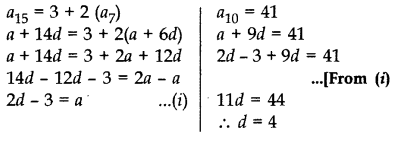From (i),
a = 2(4) – 3
= 8 – 3 = 5
nth term = a + (n – 1) d

nth term = 5 + (n – 1) 4
= 5 + 4n – 4 = (4n + 1)

The 16th term of an AP is 1 more than twice its 8th term. If the 12th term of the AP is 47, then find its nth term. (2012D)

Answer :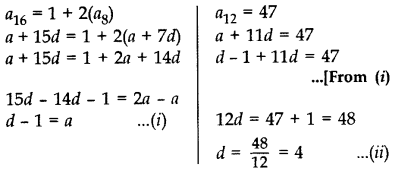From (A) and (B), a = 4 – 1 = 3
As nth term = a + (n – 1) d
nth term = 3 + (n – 1) 4
= 3 + 4n -4 = 4n -1

A sum of ₹1,600 is to be used to give ten cash prizes to students of a school for their overall academic performance. If each prize is ₹20 less than its preceding prize, find the value of each of the prizes. (2012OD)

Answer :

Here, Sum of 10 prizes = 1600

S10 = 1600, d = -20, n = 10
Sn =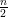(2a + (n – 1)d]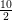[2a + (10 – 1)(-20)] = 1600
2a – 180 = 320
2a = 320 + 180 = 500
a = 250
Hence, 1st prize = a = ₹250
2nd prize = a2 = a + d = 250 + (-20) = ₹230
3rd prize = a3 = a2 + d = 230 – 20 = ₹210
4th prize = a4 = a3 + d = 210 – 20 = ₹190
5th prize = a5 = a4 + d = 190 – 20 = ₹170
6th prize = a6 = a5 + d = 170 – 20 = ₹150
7th prize = a7 = a6 + d = 150 – 20 = ₹130
8th prize = a8 = a7 + d = 130 – 20 = ₹110
9th prize = a9 = a8 + d = 110 – 20 = ₹590
10th prize = a10 = a9 + d = 90 – 20 = ₹70
= ₹ 1,600

Find the 10th term

 1 1,3,5,7…. A 57 2 2,4,6,8…. B 51 3 2,7,12,17…. C 19 4 21,24,27,30…. D 20

Answer :

1-C, 2-D, 3-A, 4-B

Find the nth term

 1 2,6,10,14…. A 8+10n 2 13,9,5,1…. B 4n-2 3 18,28,38,48…. C 26-n 4 25,24,23,22,…. D 17-4n

Answer :

1-B, 2-D, 3-A, 4-C

Find the 2nd term from last

 1 -2,5,…….,68 A 900 2 -100,0,….., 1000 B 98 3 28,30,…… , 100 C 15 4 75,45,….-15 D 61

Answer :

1-D, 2-A, 3-B, 4-C

 1 43,__, 51,55,59 A 81 2 75,78, __, 94 B 47 3 __, 27,30,33 C -164 4 82, 0, -82, __ D 24

Answer :

1-B, 2-A, 3-D, 4-C

Find the common differences

 1 89,85,81,77 A 11 2 100, 89, 78, 67 B -4 3 100, 111,122,133 C 4 4 89, 93, 97, 101 D -11

Answer :

1-B, 2-D, 3-A, 4-C

### Take a Test

Choose your Test :

### Chapter 5 : Arithmetic Progressions

In this chapter, students will discuss patterns in succeeding terms obtained by adding a fixed number to the preceding terms. They also, see how to find nth terms and the sum of n consecutive terms. Students will learn arithmetic progression effectively when they solve daily life problems.

This chapter has Arithmetic Progression Derivation of the nth term and sum of the first n terms of an A.P. and their application in solving daily life problems. This is one of the important chapters from the point of the Class 10 examination. An arithmetic progression is a very basic and important topic to study as almost all the competitive exams will ask questions on arithmetic progression.

### Browse & Download CBSE Books For Class 10 - All Subjects

The GSEB Books for class 10 are designed as per the syllabus followed Gujarat Secondary and Higher Secondary Education Board provides key detailed, and a through solutions to all the questions relating to the GSEB textbooks.

The purpose is to provide help to the students with their homework, preparing for the examinations and personal learning. These books are very helpful for the preparation of examination.

For more details about the GSEB books for Class 10, you can access the PDF which is as in the above given links for the same.